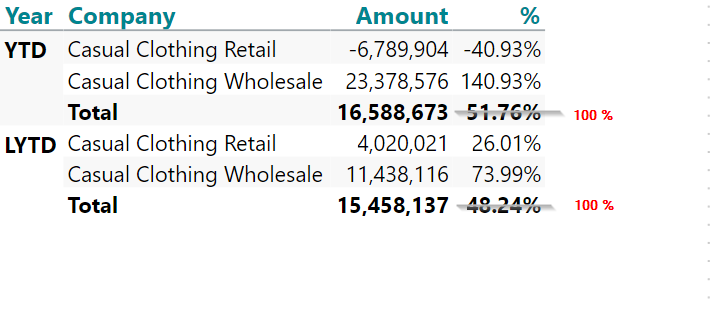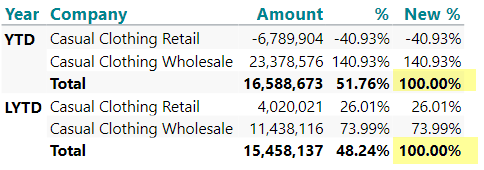# Change Total value in calculation

Hi all,

we use a calculation where the percentage should be shown per user dimension "Year".

So far so good, the formula is: sum(0, 0, m1) / sum(0, all(s), m1)

Is it possible to change the formula that auto. Totals are always 100% per user dimensions?• You need to create and extra column to my knowledge.

Assuming your % calculations is a column, I would do this and then just hide your first column.

`if allcount(c1,all(c),0) = 0 then sum(c1,0,0) else 1 `
• Hi Kristoffer,

really good, it helped a lot.(Using calculated measure it is: if allcount(0, all(c), m2) = 0 then sum(0, 0, m2) else 1)

Thank you!